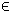## 18MINIMAX Problem

The constrained minimax (mima) problem is defined as
 min x
maxr(x)
subject to xL x xU
bL Ax bU
cL c(x) cU
(20)
where x,xL,xURn, r(x)RN, c(x),cL,cURm1, bL,bURm2 and ARm2 × n.

The following files define a problem in TOMLAB.

File: tomlab/quickguide/mimaQG_r.m, mimaQG_J.m
```  r:   Residual vector
J:   Jacobian matrix
```
The following file illustrates how to solve a minimax problem in TOMLAB. Also view the m-files specified above for more information.

File: tomlab/quickguide/minimaxQG.m

Open the file for viewing, and execute minimaxQG in Matlab.
```% minimaxQG is a small example problem for defining and solving
% minimax programming problems using the TOMLAB format.

x_0  = [1;1];        % Initial value
x_L  = [-10;-10];    % Lower bounds on x
x_U  = [10;10];      % Upper bounds on x

% Solve the problem min max |r_i(x)|, where i = 1,2,3
% Solve the problem by eliminating abs, doubling the residuals, reverse sign
% i.e. min max [r_1; r_2; r_3; -r_1; -r_2; -r_3];
y = [-1.5; -2.25; -2.625]; % The data values
y = [y ; -y];           % Eliminate abs, double the residuals, reverse sign
t = [];                 % No time vector used

%
% Add the linear constraint -x(1) + x(2) + 2 >= 0
% Write the constraint as x(1) - x(2) <= 2

% The A matrix could be specified dense or sparse
% A   = sparse([1 -1]);

A   = [1 -1];
b_L = -inf;
b_U = 2;

% Generate the problem structure using the Tomlab Quick format
%
% The part in the residuals dependent on x are defined in mima_r.m
% The Jacobian is defined in mima_J.m

Prob = clsAssign('mimaQG_r', 'mimaQG_J', [], x_L, x_U, Name, x_0, y, t, ...
[],[],[],[],A,b_L,b_U);

% Set the optimal values into the structure (for nice result presenting)

Prob.x_opt=[2.3660254038, 0.3660254038];
% Optimal residuals:
r_opt = [0; 0.2009618943;0.375];

% Compute optimal function value:
Prob.f_opt=max(abs(r_opt));

% Use the standard method in infSolve
Prob.InfType = 1;

% Get the default solver
% Solver = GetSolver('con',1,0);

Prob.SolverInf = 'conSolve';

% One may set other solvers:
%Prob.SolverInf = 'snopt';
%Prob.SolverInf = 'npsol';
%Prob.SolverInf = 'minos';
%Prob.SolverInf = 'conSolve';

% Set print level 2 to get output from PrintResult at the end
PriLev = 2;
Prob.PriLevOpt = 0;
Result  = tomRun('infSolve', Prob, PriLev);```